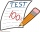# Basements

In the first basement is more flies than the spiders, the second vice versa. Each basement had spiders and flies together 100 feet. Determine how many could be flies and spiders in the first and second basement.

PS. We only need, when you write how many solutions have this problem.

Result

n =  4

#### Solution:

100 = 6m + 8p
[m; p]:

[2; 11]
[6; 8]
[10; 5]
[14; 2]

P= { {[10; 5], [2; 11]},
{[10; 5], [6; 8]},
{[14; 2], [2; 11]},
{[14; 2], [6; 8]}}

Leave us a comment of example and its solution (i.e. if it is still somewhat unclear...):Be the first to comment!#### To solve this example are needed these knowledge from mathematics:

Do you solve Diofant problems and looking for a calculator of Diofant integer equations? Do you have a linear equation or system of equations and looking for its solution? Or do you have quadratic equation?

## Next similar examples:

1. NormThree workers planted 3555 seedlings of tomatoes in one dey. First worked at the standard norm, the second planted 120 seedlings more and the third 135 seedlings more than the first worker. How many seedlings were standard norm?
2. Number with onesThe first digit of the number is 1, if we move this digit to the end we get a 3 times higher number, which is the number?
3. Unknown numberIdentify unknown number which 1/5 is 40 greater than one tenth of that number.
4. Tickets 3A total of 645 tickets were sold for the school play. They were either adult tickets or student tickets. There were 55 fewer student tickets sold than adult tickets. How many adult tickets were sold?
5. Digit sumDetermine for how many integers greater than 900 and less than 1,001 has digit sum digit of the digit sum number 1.
6. Street numbersLada came to aunt. On the way he noticed that the houses on the left side of the street have odd numbers on the right side and even numbers. The street where he lives aunt, there are 5 houses with an even number, which contains at least one digit number 6.
7. Unknown number 11That number increased by three equals three times itself?
8. EquationSolve the equation: 1/2-2/8 = 1/10; Write the result as a decimal number.
9. One-thirdA one-third of unknown number is equal to five times as great as the difference of the same unknown number and number 28. Determine the unknown number.
10. Simple equationSolve the following simple equation: 2. (4x + 3) = 2-5. (1-x)
11. Divisibility 2How many divisors has integer number 13?
12. Last pageTwo consecutive sheets dropped out of the book. The sum of the numbers on the sides of the dropped sheets is 154. What is the number of the last page of the dropped sheets?
13. Equation?
14. Examination 2In an examination, a teacher awards 2 marks for every correct answer and subtract a mark for every wrong answer. If there are 20 questions and a child scored 25 marks how many correct answer did the child get?
15. CakesGrandmother baked cakes. Half of its was poppy, quarter with plum jam and 16 cheesecakes. How many cakes she baked in total?
16. Dropped sheetsThree consecutive sheets dropped from the book. The sum of the numbers on the pages of the dropped sheets is 273. What number has the last page of the dropped sheets?
17. Write decimalsWrite in the decimal system the short and advanced form of these numbers: a) four thousand seventy-nine b) five hundred and one thousand six hundred and ten c) nine million twenty-six